Home MATHEMATICS TOPIC 9: RATIO, PROFIT AND LOSS ~ MATHEMATICS FORM 1

# TOPIC 9: RATIO, PROFIT AND LOSS ~ MATHEMATICS FORM 1

1837
2
SHAREContents

## TOPIC 9: RATIO, PROFIT AND LOSS

Ratio

A ratio – is a way of comparing quantities measured in the same units

Examples of ratios

A class has 45 girls and 40 boys. The ratio of number of boys to the number of girls = 40: 45

A football ground 100​​ 𝑚​​ long and 50​​ 𝑚​​ wide. The ratio of length to the width = 100: 50

#### NOTE:​​

Ratios can be simplified like fractions

40: 45 = 8: 9

100: 50 = 2: 1

#### A Ratio in its Simplest Form

Express a ratio in its simplest form

Example 1

Simplify the following ratios, giving answers as whole numbers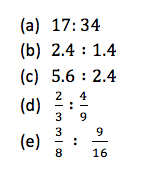Solution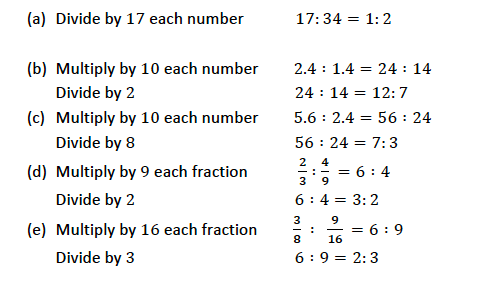A Given Quantity into Proportional Parts

Divide a given quantity into proportional parts

Example 2

Express the following ratios in the form of​​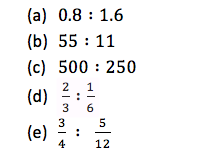Solution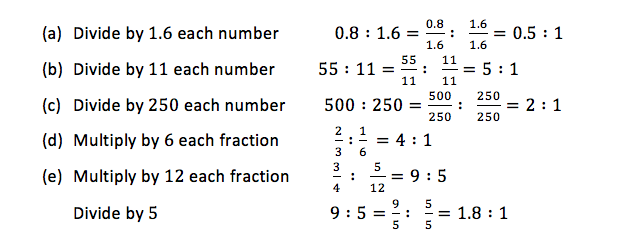To increase or decrease a certain quantity in a given ratio, multiply the quantity with that ratio

Example 3

Increase 6​​ 𝑚​​ in the ratio 4​​ ​​ 3

Decrease 800 /− in the ratio 4​​ ​​ 5

Solution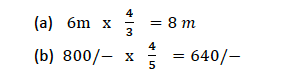#### Profits and Loss

Profit or Loss

Find profit or loss

If you buy something and then sell it at a higher price, then you have a profit which is given by: Profit = selling price − buying price

See also  TOPIC 7: TRIGONOMETRY(II) ~ ADV MATHEMATICS FORM 5

If you buy something and then sell it at a lower price, then you have a loss which is given by: Loss = buying price − selling price

The profit or loss can also be expressed as a percentage of buying price as follows: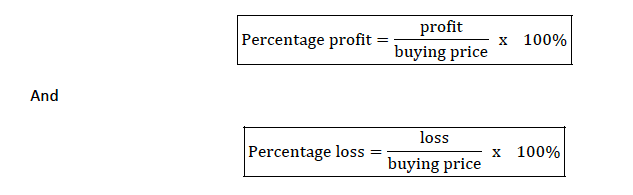Percentage Profit and Percentage Loss

Calculate percentage profit and percentage profit and percentage loss

Example 4

Mr. Richard bought a car for 3, 000, 000/− and sold for 3, 500, 000/−. What is the profit and percentage profit obtained?

Solution

Profit= selling price − buying price = 3,500,000-3,000,000=500,000

Therefore the profit obtained is 500,000/-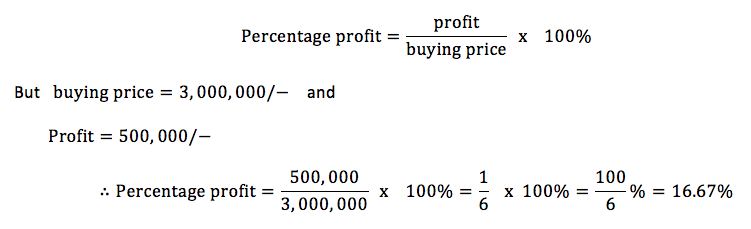Example 5

Eradia bought a laptop for​​

Solution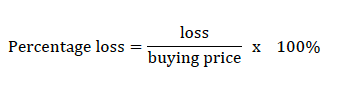But buying price = 780, 000/− and loss = buying price − selling price = 780, 000 − 720, 000 = 60, 000/−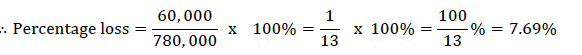#### Simple Interest

Simple Interest

Calculate simple interest

The amount of money charged when a person borrows money e. g from a bank is called interest (I)

The amount of money borrowed is called principle (P)

To calculate interest, we use interest rate (R) given as a percentage and is usually taken per year or per annum (p.a)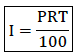Example 6

Calculate the simple interest charged on the following

850, 000/− at 15% per annum for 9 months

200, 000/− at 8% per annum for 2 years

Solution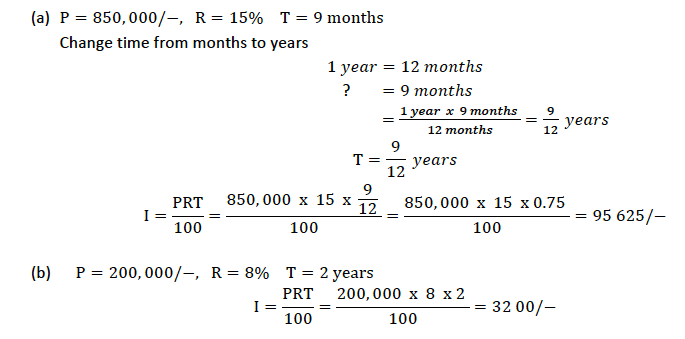Real Life Problems Related to Simple Interest

See also  TOPIC 7: GEOMETRIC AND TRANSFORMATIONS ~ MATHEMATICS FORM 2

Solve real life problems related to simple interest

Example 7

Mrs. Mihambo deposited money in CRDB bank for 3 years and 4 months. A t the end of this time she earned a simple interest of 87, 750/− at 4.5% per annum. How much had she deposited in the bank?

Solution

Given I = 87, 750/− R = 4.5% % T = 3 years and 4 months

Change months to years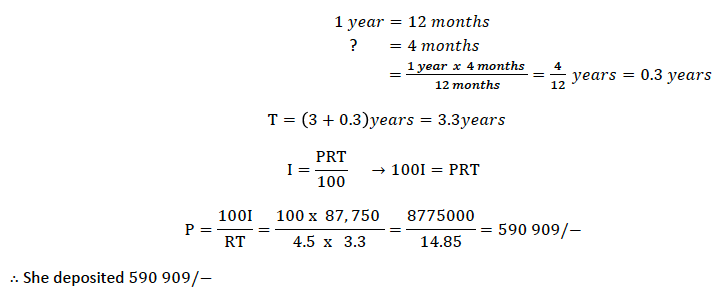1.RobertRaisp
2.MonteDig